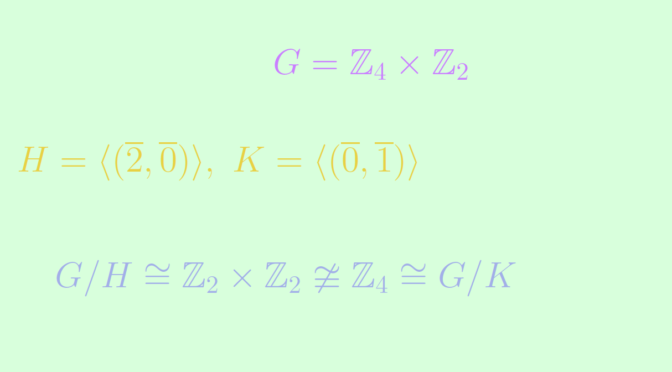# Isomorphism of factors does not imply isomorphism of quotient groups

Let $$G$$ be a group and $$H, K$$ two isomorphic subgroups. We provide an example where the quotient groups $$G / H$$ and $$G / K$$ are not isomorphic.

Let $$G = \mathbb{Z}_4 \times \mathbb{Z}_2$$, with $$H = \langle (\overline{2}, \overline{0}) \rangle$$ and $$K = \langle (\overline{0}, \overline{1}) \rangle$$. We have $H \cong K \cong \mathbb{Z}_2.$ The left cosets of $$H$$ in $$G$$ are $G / H=\{(\overline{0}, \overline{0}) + H, (\overline{1}, \overline{0}) + H, (\overline{0}, \overline{1}) + H, (\overline{1}, \overline{1}) + H\},$ a group having $$4$$ elements and for all elements $$x \in G/H$$, one can verify that $$2x = H$$. Hence $$G / H \cong \mathbb{Z}_2 \times \mathbb{Z}_2$$. The left cosets of $$K$$ in $$G$$ are $G / K=\{(\overline{0}, \overline{0}) + K, (\overline{1}, \overline{0}) + K, (\overline{2}, \overline{0}) + K, (\overline{3}, \overline{0}) + K\},$ which is a cyclic group of order $$4$$ isomorphic to $$\mathbb{Z}_4$$. We finally get the desired conclusion $G / H \cong \mathbb{Z}_2 \times \mathbb{Z}_2 \ncong \mathbb{Z}_4 \cong G / K.$